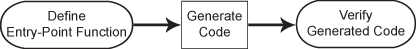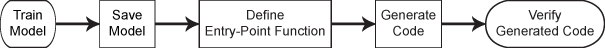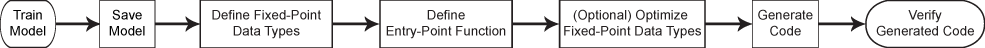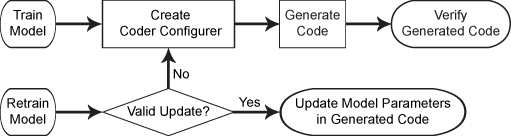## Introduction to Code Generation

MATLAB® Coder™ generates readable and portable C and C++ code from Statistics and Machine Learning Toolbox™ functions that support code generation. You can integrate the generated code into your projects as source code, static libraries, or dynamic libraries. You can also use the generated code within the MATLAB environment to accelerate computationally intensive portions of your MATLAB code.

Generating C/C++ code requires MATLAB Coder and has the following limitations:

• You cannot call any function at the top level when generating code by using `codegen`. Instead, call the function within an entry-point function, and then generate code from the entry-point function. The entry-point function, also known as the top-level or primary function, is a function you define for code generation. All functions within the entry-point function must support code generation.

• The MATLAB Coder limitations also apply to Statistics and Machine Learning Toolbox for code generation. For details, see MATLAB Language Features Supported for C/C++ Code Generation (MATLAB Coder).

• Code generation in Statistics and Machine Learning Toolbox does not support sparse matrices and categorical arrays. Code generation supports numeric tables for most prediction functions.

• For the code generation usage notes and limitations for each function, see the Code Generation section on the function reference page.

For a list of Statistics and Machine Learning Toolbox functions that support code generation, see Function List (C/C++ Code Generation).

### Code Generation Workflows

You can generate C/C++ code for the Statistics and Machine Learning Toolbox functions in several ways.

• General code generation workflow for functions that are not the object functions of machine learning modelsDefine an entry-point function that calls the function that supports code generation, generate C/C++ code for the entry-point function by using `codegen`, and then verify the generated code. The entry-point function, also known as the top-level or primary function, is a function you define for code generation. Because you cannot call any function at the top level using `codegen`, you must define an entry-point function. All functions within the entry-point function must support code generation.

For details, see General Code Generation Workflow.

• Code generation workflow for the object function (`predict`, `random`, `knnsearch`, or `rangesearch`) of a machine learning modelSave a trained model by using `saveLearnerForCoder`, and define an entry-point function that loads the saved model by using `loadLearnerForCoder` and calls the object function. Then generate code for the entry-point function by using `codegen`, and verify the generated code. The input arguments of the entry-point function cannot be classification or regression model objects. Therefore, you need to work around this limitation by using `saveLearnerForCoder` and `loadLearnerForCoder`.

For details, see these examples

You can also generate fixed-point C/C++ code for the prediction of a support vector machine (SVM) model, a decision tree model, and an ensemble of decision trees for classification and regression. This type of code generation requires Fixed-Point Designer™.Fixed-point code generation requires an additional step that defines the fixed-point data types of the variables required for prediction. Create a fixed-point data type structure by using the data type function generated by `generateLearnerDataTypeFcn`, and use the structure as an input argument of `loadLearnerForCoder` in an entry-point function. You can also optimize the fixed-point data types before generating code.

For details, see Fixed-Point Code Generation for Prediction of SVM.

• Code generation workflow for the `predict` and `update` functions of a tree model, an SVM model, a linear model, or a multiclass error-correcting output codes (ECOC) classification model using SVM or linear binary learnersCreate a coder configurer by using `learnerCoderConfigurer`, generate code by using `generateCode`, and then verify the generated code. You can configure code generation options and specify the coder attributes of the model parameters using object properties. After you retrain the model with new data or settings, you can update model parameters in the generated C/C++ code without having to regenerate the code. This feature reduces the effort required to regenerate, redeploy, and reverify C/C++ code.

For details, see Code Generation for Prediction and Update Using Coder Configurer.

### Code Generation Applications

Code generation for the Statistics and Machine Learning Toolbox functions also works with other toolboxes such as Simulink®, System object™, and Stateflow®, as described in these examples:

For more applications of code generation, see these examples: